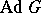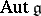# Difference between revisions of "Adjoint group"

Jump to: navigation, search

of a groupThe linear groupthat is the image of the Lie group or algebraic groupunder the adjoint representation (cf. Adjoint representation of a Lie group). The adjoint groupis contained in the groupof automorphisms of the Lie algebraof, and its Lie algebra coincides with the adjoint algebraof. A connected semi-simple group is a group of adjoint type (i.e. is isomorphic to its adjoint group) if and only if its roots generate the lattice of rational characters of the maximal torus; the centre of such a group is trivial. If the ground field has characteristic zero andis connected, thenis uniquely determined by the Lie algebraand is either called the adjoint group or the group of inner automorphisms of. In particular, ifis semi-simple,coincides with the connected component of the identity in.

How to Cite This Entry:
Adjoint group. Encyclopedia of Mathematics. URL: http://encyclopediaofmath.org/index.php?title=Adjoint_group&oldid=21786
This article was adapted from an original article by A.L. Onishchik (originator), which appeared in Encyclopedia of Mathematics - ISBN 1402006098. See original article